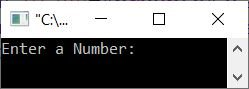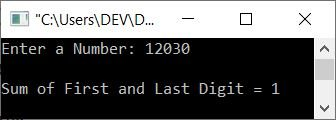# C++ program to find the sum of the first and last digits of a number

This article is created to provide some programs in C++ to find and print the sum of the first and last digits of a given number. The program is created in the following ways:

## Using a while loop, calculate the sum of the first and last digits

The question is: write a program in C++ that receives a number from the user as input and prints the sum of the first and last digits of the given number as output. Here is its answer:

```#include<iostream>

using namespace std;
int main()
{
int num, temp=0, last, rem, sum=0;
cout<<"Enter a Number: ";
cin>>num;
while(num!=0)
{
if(temp==0)
{
last = num%10;
temp++;
}
rem = num%10;
num = num/10;
}
sum = rem + last;
cout<<"\nSum of First ("<<rem<<") and Last ("<<last<<") Digit = "<<sum;
cout<<endl;
return 0;
}```

The snapshot given below shows the initial output produced by the above C++ program on finding the sum of the first and last digits of a given number:Now supply the number, say 23094, as input and press the ENTER key to find and print the sum of the first (that is  2) and last (that is 4) digits of this number, like shown in the snapshot given below:In the above program, the dry run of the following block of code

```   while(num!=0)
{
if(temp==0)
{
last = num%10;
temp++;
}
rem = num%10;
num = num/10;
}```

It goes like this with num=23094, temp=0, and sum=0:

• The condition num!=0 or 23094!=0 is satisfied.
• Therefore, program flow goes inside the loop, and the condition of the if gets evaluated.
• That is, the condition temp==0 or 0==0 evaluates to true.
• As a result, program flow enters the body of if, and num%10 or 23094%10 or 4 is set to last.
• And the value of temp is incremented. So temp=1. Because the value of temp is 1 from now, the condition of if always gets evaluated to be false from now.
• This means the variable last holds 4 as its final value. That is the last digit of the given number.
• After exiting the if, num%10, 23094%10, or 4 is initialized to rem. This statement is created to get the last remainder, which is 2 at last execution.
• And the loop's final statement is executed, num/10 or 23094/10 or 2309 is initialized to num. As a result, num=2309 now.
• Since all statements available in the body of the while loop get executed, the program flow evaluates its condition again.
• That is, the condition num!=0 or 2309!=0 evaluates to true once more. Therefore, program flow again goes inside the loop.
• This process continues, until the condition is evaluated as false.
• In this way, after exiting from the loop, we will have last = 4 and rem = 2.
• Therefore, just add them and print the addition result of the first and last digits of the given number. That's it

## Using a for loop, calculate the sum of the first and last digits

Since the for loop also contains initialize and update statements along with condition checking, Therefore, just place any initialization, say temp = 0 or sum = 0, and the update statement as num = num/10, like shown in the program given below:

```#include<iostream>

using namespace std;
int main()
{
int num, temp, last, rem, sum=0;
cout<<"Enter a Number: ";
cin>>num;
for(temp=0; num!=0; num=num/10)
{
if(temp==0)
{
last = num%10;
temp++;
}
rem = num%10;
}
sum = rem + last;
cout<<"\nSum of First ("<<rem<<") and Last ("<<last<<") Digit = "<<sum;
cout<<endl;
return 0;
}```

This program exactly produces the same output as the previous program.

## Using Function, compute the sum of the first and last digits

This is the last program of this article, created using a user-defined function named sumfun() that takes the given number as its argument and returns the sum of the first and last digits of its argument.

```#include<iostream>
using namespace std;

int sumfun(int);
int main()
{
int num, sum;
cout<<"Enter a Number: ";
cin>>num;
sum = sumfun(num);
cout<<"\nSum of First and Last Digit = "<<sum;
cout<<endl;
return 0;
}
int sumfun(int n)
{
int t=0, l, r, s=0;
while(n!=0)
{
if(t==0)
{
l = n%10;
t++;
}
r = n%10;
n = n/10;
}
s = r + l;
return s;
}```

Here is its sample run with user input 12030:C++ Quiz

« Previous Program Next Program »